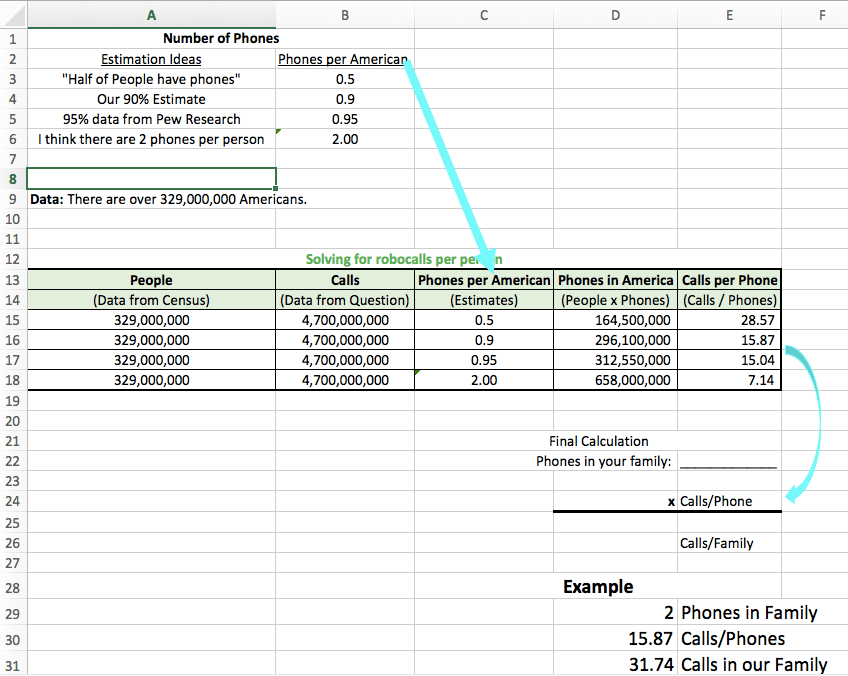Solutions to June 9 Math Question

Here is one solution that uses a spreadsheet to compare different estimation strategies.The first table shows different ways we could estimate (or research) the number of phones per person. Then, we rounded the Census data to create a table to calculate the number of calls for each estimate. In the fourth column, we multiplied the number of People by the estimated Phones per Americans. In the last column, we divided the Number of Calls by the Number of Phones to get the average number of calls each phone receives.

This photo shows how we worked it out initially.